/

### The Frank copula

The Frank copula is a copula that is sometimes used in the modelling of codependency. It is an Archimedean copula, and exchangeable.

 Copula name Frank copula Common notation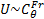Parameters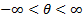Domain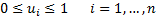Copula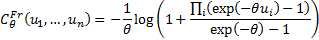Or if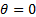we use the limit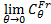which the independence copula Kendall’s rank correlation coefficient (for bivariate case)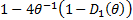where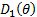is the Debye function defined as:Coefficient of upper tail dependence,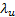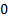Coefficient of lower tail dependence,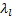Archimedean generator function,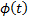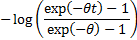Or ifwe use the limit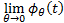which is taken as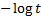Other comments Ifwe obtain the independence copula. The Frank copula (like the Clayton copula) is a comprehensive copula in that it interpolates between a lower limit of the countermonotonicity copula (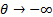) and an upper limit of the comonotonicity copula (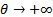).

Nematrian web functions

Functions relating to the above distribution may be accessed via the Nematrian web function library by using a DistributionName of “Frank Copula”. For details of other supported probability distributions see here.